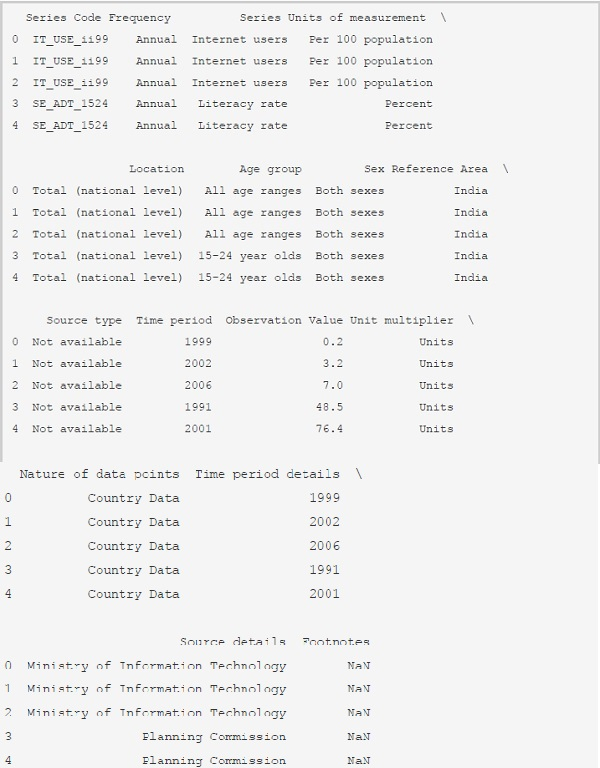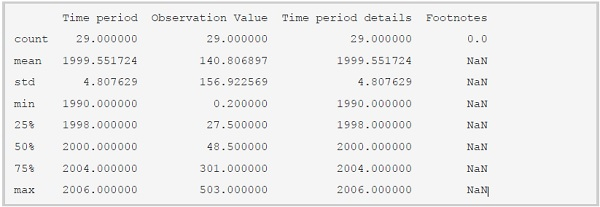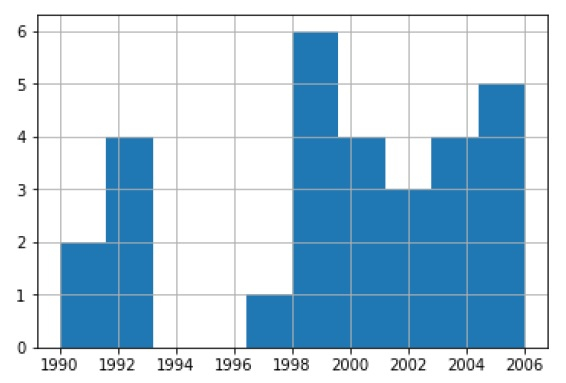# Data analysis and Visualization with Python program

PythonServer Side ProgrammingProgramming

In this tutorial, we are going to learn about data analysis and visualization using modules like pandas and matplotlib in Python. Python is an excellent fit for the data analysis things. Install the modules pandas and matplotlib using the following commands.

pip install pandas

pip install matplotlib

You will get a success message after the completion of the installation process. We will first learn about the pandas and then will see matplotlib.

## pandas

Pandas is an open-source library of Python which provides data analysis tools. We are going to see some useful methods from the pandas for data analysis.

### Creating DataFrames

We need multiple rows to create a DataFrame. Let's see how to do it.

#### Example

# importing the pands package
import pandas as pd
# creating rows
hafeez = ['Hafeez', 19]
aslan = ['Aslan', 21]
kareem = ['Kareem', 18]
# pass those Series to the DataFrame
# passing columns as well
data_frame = pd.DataFrame([hafeez, aslan, kareem], columns = ['Name', 'Age'])
# displaying the DataFrame
print(data_frame)

#### Output

If you run the above program, you will get the following results.

Name Age
0 Hafeez 19
1 Aslan 21
2 Kareem 18

### Importing Data Using pandas

Go to the link and download CSV file. The data in the CSV will be in rows with a comma(,) separated. Let's see how to import and use the data using pandas.

#### Example

# importing pandas package
import pandas as pd
# importing the data using pd.read_csv() method
# displaying the first 5 rows using data.head() method
print(data.head())

#### Output

If you run the above program, you will get the following results.Let's see how many rows and columns are there using the shape variable.

#### Example

# importing pandas package
import pandas as pd
# importing the data using pd.read_csv() method
# no. of rows and columns
print(data.shape)

#### Output

If you run the above program, you will get the following results.

(29, 16)

We have a method called describe() which computes various statistics excluding NaN. Let's see it once.

#### Example

# importing pandas package
import pandas as pd
# importing the data using pd.read_csv() method
# no. of rows and columns
print(data.describe())

#### Output

If you run the above program, you will get the following results.### Data Plotting

We have package matplotlib to create graphs using the data. Let's see how to create various types of graphs using matplotlib.

#### Example

# importing the pyplot module to create graphs
import matplotlib.pyplot as plot
# importing the data using pd.read_csv() method
# creating a histogram of Time period
data['Time period'].hist(bins = 10)

#### Output

If you run the above program, you will get the following results.

<matplotlib.axes._subplots.AxesSubplot at 0x25e363ea8d0>We can create different types of graphs using the matplotlib package.

## Conclusion

If you have any doubts regarding the tutorial, mention them in the comment section.